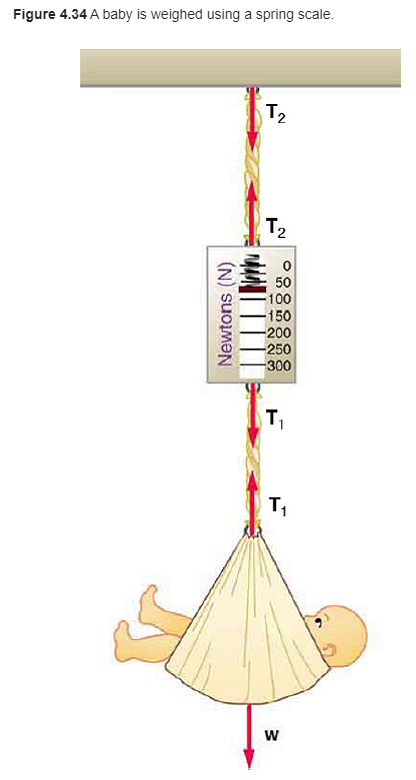# Problem: Consider the baby being weighed in Figure 4.34. (a) What is the mass of the child and basket if a scale reading of 55 N is observed? (b) What is the tension T1 in the cord attaching the baby to the scale? (c) What is the tension T2 in the cord attaching the scale to the ceiling, if the scale has a mass of 0.500 k? )d) Draw a sketch of the situation indicating the system of interest used to solve each part. The masses of the cords are negligible.

###### FREE Expert Solution

Weight:

$\overline{){\mathbf{w}}{\mathbf{=}}{\mathbf{m}}{\mathbf{g}}}$

(a)

From the equation for weight, mass is given by:

m = w/g

94% (302 ratings)###### Problem Details

Consider the baby being weighed in Figure 4.34. (a) What is the mass of the child and basket if a scale reading of 55 N is observed? (b) What is the tension T1 in the cord attaching the baby to the scale? (c) What is the tension T2 in the cord attaching the scale to the ceiling, if the scale has a mass of 0.500 k? )d) Draw a sketch of the situation indicating the system of interest used to solve each part. The masses of the cords are negligible.